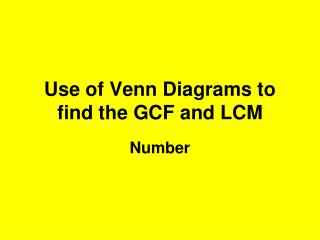DownloadDownload PresentationUse of Venn Diagrams to find the GCF and LCM

# Use of Venn Diagrams to find the GCF and LCM

Download Presentation## Use of Venn Diagrams to find the GCF and LCM

- - - - - - - - - - - - - - - - - - - - - - - - - - - E N D - - - - - - - - - - - - - - - - - - - - - - - - - - -
##### Presentation Transcript

1. Find the HCF and LCM of 24 and 60 • Use a factor tree to help you find the Prime Factors • Then a Venn Diagram to help you find the GCF and LCM • Can you remember how to draw a Factor Tree?

2. FACTOR TREES 24 60 4 15 6 4 2 2 2 3 2 2 3 5 Prime Factors have now been found.

3. Prime Factors • 24 = 2 x 2 x 2 x 3 • 60 = 2 x 2 x 3 x 5 We now need to find the GCF - Greatest Common Factor and the LCM - Lowest Common Multiple

4. Venn Diagram GCF is the region of intersection 2 x 2 x 3 = 12 24 = 2 x 2 x 2 x 3 60 = 2 x 2 x 3 x 5 60 24 2 2 5 2 LCM is all the numbers in the circles multiplied together 2 x 2 x 2 x 3 x 5=120 3 HCF = 2 x 2 x 3 = 12 LCM = 2 x 2 x 2 x 3 x 5 = 120

5. The LCM of two or more numbers is the smallest number that is a multiple of each of the numbers. 24 x 5 =120 60 x 2 =120 or you could write out the multiples of24 and 60 24, 48, 72, 96, 120, 144,…. 60, 120, 180, 240, 300,.... The GCF of two or more numbers is the largest number that divides exactly into each of them. 24 ÷ 12 = 2 60 ÷ 12 = 5

6. Can you do some questions now on your own?

7. Find the GCF and LCM of the following: • 6 and 15 • 20 and 30 • 14 and 42 • 24, 30 and 54 • Answers: • GCF = 3, LCM = 30 • GCF = 10, LCM = 60 • GCF = 14, LCM = 42 • GCF = 6, LCM = 1080